$$\newcommand{\id}{\mathrm{id}}$$ $$\newcommand{\Span}{\mathrm{span}}$$ $$\newcommand{\kernel}{\mathrm{null}\,}$$ $$\newcommand{\range}{\mathrm{range}\,}$$ $$\newcommand{\RealPart}{\mathrm{Re}}$$ $$\newcommand{\ImaginaryPart}{\mathrm{Im}}$$ $$\newcommand{\Argument}{\mathrm{Arg}}$$ $$\newcommand{\norm}{\| #1 \|}$$ $$\newcommand{\inner}{\langle #1, #2 \rangle}$$ $$\newcommand{\Span}{\mathrm{span}}$$

# 9.E: Applications of Curves in a Plane (Exercises)

$$\newcommand{\vecs}{\overset { \rightharpoonup} {\mathbf{#1}} }$$ $$\newcommand{\vecd}{\overset{-\!-\!\rightharpoonup}{\vphantom{a}\smash {#1}}}$$$$\newcommand{\id}{\mathrm{id}}$$ $$\newcommand{\Span}{\mathrm{span}}$$ $$\newcommand{\kernel}{\mathrm{null}\,}$$ $$\newcommand{\range}{\mathrm{range}\,}$$ $$\newcommand{\RealPart}{\mathrm{Re}}$$ $$\newcommand{\ImaginaryPart}{\mathrm{Im}}$$ $$\newcommand{\Argument}{\mathrm{Arg}}$$ $$\newcommand{\norm}{\| #1 \|}$$ $$\newcommand{\inner}{\langle #1, #2 \rangle}$$ $$\newcommand{\Span}{\mathrm{span}}$$ $$\newcommand{\id}{\mathrm{id}}$$ $$\newcommand{\Span}{\mathrm{span}}$$ $$\newcommand{\kernel}{\mathrm{null}\,}$$ $$\newcommand{\range}{\mathrm{range}\,}$$ $$\newcommand{\RealPart}{\mathrm{Re}}$$ $$\newcommand{\ImaginaryPart}{\mathrm{Im}}$$ $$\newcommand{\Argument}{\mathrm{Arg}}$$ $$\newcommand{\norm}{\| #1 \|}$$ $$\newcommand{\inner}{\langle #1, #2 \rangle}$$ $$\newcommand{\Span}{\mathrm{span}}$$

## 9.1: Conic Sections

### Terms and Concepts

1. What is the difference between degenerate and nondegenerate conics?

2. Use your own words to explain what the eccentricity of an ellipse measures.

3. What has the largest eccentricity: an ellipse or a hyperbola?

4. Explain why the following is true: “If the coefficient of the $$x^2$$ term in the equation of an ellipse in standard form is smaller than the coefficient of the $$y^2$$ term, then the ellipse has a horizontal major axis.”

5. Explain how one can quickly look at the equation of a hyperbola in standard form and determine whether the transverse axis is horizontal or vertical.

### Problems

In Exercises 6-13, find the equation of the parabola defined by the given information. Sketch the parabola.

6. Focus: $$(3,2)$$; directrix: $$y=1$$

7. Focus: $$(-1,-4)$$; directrix: $$y=2$$

8. Focus: $$(1,5)$$; directrix: $$x=3$$

9. Focus: $$(1/4,0)$$; directrix: $$x=-1/4$$

10. Focus: $$(1,1)$$; vertex: $$(1,2)$$

11. Focus: $$(-3,0)$$; vertex: $$(0,0)$$

12. Vertex: $$(0,0)$$; directrix: $$y=-1/16$$

13. Vertex: $$(2,3)$$; directrix: $$x=4$$

In Exercises 14-15, the equation of a parabola and a point on its graph are given. Find the focus and directrix of the parabola, and verify that the given point is equidistant from the focus and directrix.

14. $$y=\frac{1}{4}x^2,\,P=(2,1)$$

15. $$x=\frac{1}{8}(y-2)^2+3,\,P=(11,10)$$

In Exercises 16-17, sketch the ellipse defined by the given equation. Label the center, foci and vertices.

16. $$\frac{(x-1)^2}{3}+\frac{(y-2)^2}{5}=1$$

17. $$\frac{1}{25}x^2+\frac{1}{9}(y+3)^2=1$$

In Exercises 18-19, find the equation of the ellipse shown in the graph. Give the location of the foci and the eccentricity of the ellipse.

18.19.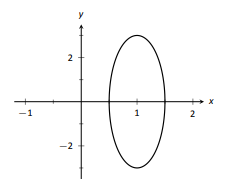In Exercises 20-23, find the equation of the ellipse defined by the given information. Sketch the ellipse.

20. Foci: $$(\pm 2,0)$$; vertices: $$(\pm 3,0)$$

21. Foci: $$(- 1,3)\text{ and }(5,3)$$; vertices: $$(-3,3)\text{ and }(7,3)$$

22. Foci: $$(2,\pm 2)$$; vertices: $$(2,\pm 7)$$

23. Focus: $$(-1,5)$$; vertex: $$(-1,-4)$$; center: $$(-1,1)$$

In Exercises 24-27, write the equation of the given ellipse in standard form.

24. $$x^2-2x+2y^2-8y=-7$$

25. $$5x^2+3y^2=15$$

26. $$3x^2+2y^2-12y+6=0$$

27. $$x^2+y^2-4x-4y+4=0$$

28. Consider the ellipse given by $$\frac{(x-1)^2}{4}+\frac{(y-3)^2}{12}=1$$.
(a) Verify that the foci are located at $$(1,3\pm 2\sqrt{2})$$
(b) The point $$P_1 = (2,6)\text{ and }P_2 = (1+\sqrt{2},3+\sqrt{6})\approx (2.414,5.449)$$ lie on the ellipse. Verify that the sum of distances from each point to the foci is the same.

In Exercises 29-32, find the equation of the hyperbola shown in the graph.

29.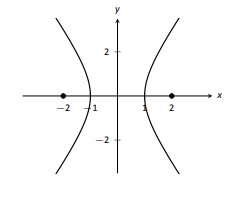30.31.32.In Exercises 33-34, sketch the hyperbola defined by the given equation. Label the center and foci.

33. $$\frac{(x-1)^2}{16}-\frac{(y+2)^2}{9}=1$$

34. $$(y-4)^2-\frac{(x+1)^2}{25}=1$$

In Exercises 35-38, find the equation of the hyperbola defined by the given information. Sketch the hyperbola.

35. Foci: $$(\pm 3,0);$$ vertices: $$(\pm 2, 0)$$

36. Foci: $$(0, \pm 3);$$ vertices: $$(0, \pm 2)$$

37. Foci: $$(-2,3)\text{ and }(8,3)$$ vertices: $$(-1,3)\text{ and }(7,3)$$

38. Foci: $$(3,-2)\text{ and }(3,8)$$ vertices: $$(3,0)\text{ and }(3,6)$$

In Exercises 39-42, write the equation of the hyperbola in standard form.

39. $$3x^2-4y^2=12$$

40. $$3x^2-y^2+2y=10$$

41. $$x^2-10y^2+40y=30$$

42. $$(4y-x)(4y+x)=4$$

43. Johannes Kepler discovered that the planets of our solar system have elliptical orbits with the Sun at one focus. The Earth's elliptical orbit is used as a standard unit of distance; the distance from the center of Earth's elliptical orbit to one vertex is 1 Astronomical Unit, or A.U.
The following table gives information about the orbits of three planets.(a) In an ellipse, knowing $$c^2=a^2-b^2\text{ and }e=c/a$$ allows us to find b in terms of a and e. Show $$b=a\sqrt{1-e^2}$$.
(b) For each planet, find equations of their elliptical orbit of the form $$\frac{x^2}{a^2}+\frac{y^2}{b^2}=1$$. (This places the center at (0,0), but the Sun is in a different location for each planet.)
(c) Shift the equations so that the Sun lies at the origin. Plot the three elliptical orbits.

44. A loud sound is recorded at three stations that lie on a line as shown in the figure below. Station A recorded the sound 1 second after Station B, and Station C recorded the sound 3 seconds after B. Using the speed of sound as 340m/s, determine the location of the sound's origination.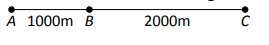## 9.2: Parametric Equations

### Terms and Concepts

1. T/F: When sketching the graph of parametric equations, the x and y values are found separately, then plotted together.

2. The direction in which a graph is "moving" is called the _____ of the graph.

3. An Equation written as $$y=f(x)$$ is written in ____ form.

4. Create parametric equations $$x=f(t),\,y=g(t)$$ and sketch their graph. Explain any interesting features of your graph based on the functions $$f$$ and $$g$$.

### Problems

In Exercises 5-8, sketch the graph of the given parametric equations by hand, making a table of points to plot. Be sure to indicate the orientation of the graph.

5. $$x=t^2+t,\quad y=1-t^2,\quad -3\le t\le 3$$

6. $$x=1,\quad y=5\sin t,\quad -\pi/2\le t\le \pi/2$$

7. $$x=t^2,\quad y=2,\quad -2\le t\le 2$$

8. $$x=t^3-t+3,\quad y=t^2+1,\quad -2\le t\le 2$$

In Exercises 9-17, sketch the graph of the given parametric equation; using a graphing utility is advisable. Be sure to indicate the orientation of the graph.

9. $$x=t^3-2t^2,\quad y=t^2,\quad -2\le t\le 3$$

10. $$x=1/t,\quad y=\sin t,\quad 0\le t\le 10$$

11. $$x=3\cos t,\quad y=5\sin t,\quad 0\le t\le 2\pi$$

12. $$x=3\cos t,\quad y=5\sin t+3,\quad 0\le t\le 2\pi$$

13. $$x=\cos t,\quad y=\cos (2t),\quad 0\le t\le \pi$$

14. $$x=\cos t,\quad y=\sin (2t),\quad 0\le t\le 2\pi$$

15. $$x=2\sec t,\quad y=3\tan t,\quad -\pi/2 \le t\le \pi/2$$

16. $$x=\cos t +\frac{1}{4}\cos (8t),\quad y=\sin t +\frac{1}{4}\sin (8t),\quad 0\le t\le 2\pi$$

17. $$x=\cos t +\frac{1}{4}\sin (8t),\quad y=\sin t +\frac{1}{4}\cos (8t),\quad 0\le t\le 2\pi$$

In Exercises 18-19, four sets of parametric equations are given. Describe how their graphs are similar and different. Be sure to discuss orientation and ranges.

18.
(a) $$x=t\quad y=t^2,\quad -\infty < t <\infty$$
(b) $$x=\sin t\quad y=\sin^2 t,\quad -\infty < t <\infty$$
(c) $$x=e^t\quad y=e^{2t},\quad -\infty < t <\infty$$
(d) $$x=-t\quad y=t^2,\quad -\infty < t <\infty$$

19.
(a) $$x=\cos t\quad y=\sin t,\quad 0\le t \le 2\pi$$
(b) $$x=\cos (t^2)\quad y=\sin (t^2),\quad 0\le t \le 2\pi$$
(c) $$x=\cos (1/t)\quad y=\sin (1/t),\quad 0\le t \le 2\pi$$
(d) $$x=\cos (\cos t)\quad y=\sin (\cos t),\quad 0\le t \le 2\pi$$

In Exercises 20-29, eliminate the parameter in the given parametric equations.

20. $$x=2t+5,\quad y=-3t+1$$

21. $$x=\sec t,\quad y=\tan t$$

22. $$x=4\sin t +1,\quad y=3\cos t-2$$

23. $$x=t^2,\quad y=t^3$$

24. $$x=\frac{1}{t+1},\quad y=\frac{3t+5}{t+1}$$

25. $$x=e^t,\quad y=e^{3t}-3$$

26. $$x=\ln t,\quad y=t^2-1$$

27. $$x=\cot t,\quad y=\csc t$$

28. $$x=\cosh t,\quad y=\sinh t$$

29. $$x=\cos (2t),\quad y=\sin t$$

In Exercises 30-33, eliminate the parameter in the given parametric equations. Describe the curve defined by the parametric equations based on its rectangular form.

30. $$x=at+x_0,\quad y=bt+y_0$$

31. $$x=r\cos t,\quad y=r\sin t$$

32. $$x=a\cos t +h,\quad y=b\sin t +k$$

33. $$x=a\sec t +h,\quad y=b\tan t +k$$

In Exercises 34-37, find parametric equations for the given rectangular equation using the parameter $$t=\frac{dy}{dx}$$. Verify that at $$t=1$$, the point on the graph has a tangent line with slope of 1.

34. $$y=3x^2-11x+2$$

35. $$y=e^x$$

36. $$y=\sin x \text{ on }[0,\pi]$$

37. $$y=\sqrt{x}\text{ on }[0,\pi]$$

In Exercises 42-45, find the value(s) of t where the graph of the parametric equations crosses itself.

38. $$x=t^3-t+3,\quad y=t^2-3$$

39. $$x=t^3-4t^2+t+7,\quad y=t^2-t$$

40. $$x=\cos t,\quad y=\sin (2t)\text{ on }[0,2\pi]$$

41. $$x=\cos t \cos (3t),\quad y=\sin t \cos (3t)\text{ on }[0,\pi]$$

In Exercises 42-45, find the value(s) of t where the curve defined by the parametric equations is not smooth.

42. $$x=t^3+t^2-t,\quad y=t^2+2t+3$$

43. $$x=t^2-4t,\quad y=t^3-2t^2-4t$$

44. $$x=\cos t,\quad y=2\cos t$$

45. $$x=2\cos t -\cos (2t),\quad y=2\sin t -\sin (2t)$$

In Exercises 46-54, find parametric equations that describe the given situation.

46. A projectile is fired from a height of 0ft, landing 16ft away in 4s.

47. A projectile is fired from a height of 0ft, landing 200ft away in 4s.

48. A projectile is fired from a height of 0ft, landing 200ft away in 20s.

49. A circle of radius 2, centered at the origin, that is traced clockwise once on $$[0,2\pi]$$.

50. A circle of radius 3, centered at $$(1,1)$$, that is traced once counter-clockwise once on $$[0,1]$$.

51. An ellipse centered at (1,3) with vertical major axis of length 6 and minor axis of length 2.

52. An ellipse with foci at $$(\pm 1,0)$$ and vertices at $$(\pm 5,0)$$.

53. A hyperbola with foci at $$(5,-3)$$ and $$(-1,-3)$$, and with vertices at $$(1,-3)$$ and $$(3,-3)$$.

54. A hyperbola with vertices at $$(0,\pm 6)$$ and asymptotes $$y=\pm 3x$$.

## 9.3: Calculus and Parametric Equations

### Terms and Concepts

1. T/F: Given parametric equations $$x=f(t)\text{ and }y=g(t)$$, $$\frac{dy}{dx}=f'(t)f'(t)$$, as long as $$g'(t)\ne 0$$.

2. Given parametric equations $$x=f(t)\text{ and }y=g(t)$$, the derivative $$\frac{dy}{dx}$$ as given in Key Idea 37 is a function of _________?

3. T/F: Given parametric equations $$x=f(t)\text{ and }y=g(t)$$, to find $$\frac{d^2y}{dx^2}$$, one simply computes $$\frac{d}{dt}\left (\frac{dy}{dx}\right )$$.

4. T/F: If $$\frac{dy}{dx}=0$$ at $$t=t_0$$, then the normal line to the curve at $$t=t_0$$ is a vertical line.

### Problems

In Exercises 5-12, parametric equations for a curve are given.
(a) Find
$$\frac{dy}{dx}$$.
(b) Find the equations of the tangent and normal line(s) at the point(s) given.
(c) Sketch the graph of the parametric functions along with the found tangent and normal lines.

5. $$x=t,\, y=t^2;\quad t=1$$

6. $$x=\sqrt{t},\, y=5t+2;\quad t=4$$

7. $$x=t^2-t,\, y=t^2+t;\quad t=1$$

8. $$x=t^2,\, y=t^3-1;\quad t=0 \text{ and }t=1$$

9. $$x=\sec t,\, y=\tan t \text{ on }(-\pi/2,\pi/2);\quad t=\pi/4$$

10. $$x=\cos t,\, y=\sin (2t);\quad t=\pi/4$$

11. $$x=\cos t \sin (2t),\, y=\sin t \sin (2t)\text{ on }[0,2\pi];\quad t=3\pi /4$$

12. $$x=e^{t/10},\, y=e^{t/10}\sin t;\quad t=\pi/2$$

In Exercises 13-20, find t-values where the curve defined by the given parametric equations has a horizontal tangent line. Note: these are the same equations as in Exercises 5-12.

13. $$x=t,\,y=t^2$$

14. $$x=\sqrt{t},\,y=5t+2$$

15. $$x=t^2-t,\,y=t^2+t$$

16. $$x=t^2-1,\,y=t^3-t$$

17. $$x=\sec t,\,y=\tan t \text{ on }(-\pi/2, \pi/2)$$

18. $$x=\cos t,\,y=\sin (2t)\text{ on }[0,2\pi]$$

19. $$x=\cos t\sin (2t),\,y\sin t\sin (2t)\text{ on }[0,2\pi]$$

20. $$x=e^{t/10}\cos t,\,y=e^{t/10}\sin t$$

In Exercises 21-24, find $$t=t_0$$ where the graph of the given parametric equations is not smooth, then find $$\lim\limits_{t\to t_0}\frac{dy}{dx}$$.

21. $$x=\frac{1}{t^2+1},\quad y=t^3$$

22. $$x=-t^3+7t^2-16t+13,\quad y=t^3-5t^2+8t-2$$

23. $$x=t^3-3t^2+3t-1,\quad y=t^2-2t+1$$

24. $$x=\cos^2 t,\quad y=1-\sin^2 t$$

In Exercises 25-32, parametric equations for a curve are given. Find $$\frac{d^2y}{dx^2}$$, then determine the intervals on which the graph of the curve is concave up/down. Note: these are the same equations as in Exercises 5-12.

25. $$x=t,\, y=t^2;\quad t=1$$

26. $$x=\sqrt{t},\, y=5t+2;\quad t=4$$

27. $$x=t^2-t,\, y=t^2+t;\quad t=1$$

28. $$x=t^2,\, y=t^3-1;\quad t=0 \text{ and }t=1$$

29. $$x=\sec t,\, y=\tan t \text{ on }(-\pi/2,\pi/2);\quad t=\pi/4$$

30. $$x=\cos t,\, y=\sin (2t);\quad t=\pi/4$$

31. $$x=\cos t \sin (2t),\, y=\sin t \sin (2t)\text{ on }[0,2\pi];\quad t=3\pi /4$$

32. $$x=e^{t/10},\, y=e^{t/10}\sin t;\quad t=\pi/2$$

In Exercises 37-40, numerically approximates the given arc length.

37. Approximate the arc length of one petal of the rose curve $$x=\cos t \cos (2t)$$ using Simpson's Rule and $$n=4$$.

38. Approximate the arc length of the "bow tie curve" $$x=\cos t,\quad y=\sin (2t)$$ using Simpson's Rule and $$n=6$$.

39. Approximate the arc length of the parabola $$x=t^2-t$$, $$y=t^2+t$$ on [-1,1] using Simpson's Rule and $$n=4$$.

40. A common approximate of the circumference of an ellipse given by $$x=a\cos t,\quad y=b\sin t$$ is $$C\approx 2\pi \sqrt{\frac{a^2+b^2}{2}}$$.

In Exercises 41-44, a solid of revolution is described. Find or approximate its surface area as specified.

41. Find the surface area of the sphere formed by rotating the circle $$x=2\cos t,\quad y=2\sin t$$ about:
(a) the x-axis and
(b) the y-axis.

42. Find the surface are of the torus (or "donut") formed by rotating the circle $$x=\cos t+2,\quad y=\sin t$$ about the y-axis.

43. Approximate the surface are of the solid formed by rotating the "upper right half" of the bow tie curve $$x=\cos t, y=\sin (2t)\text{ on }[0,\pi/2]$$ about the x-axis, using Simpson's Rule and $$n=4$$.

44. Approximate the surface area of the solid formed by rotating the one petal of the rose curve $$x=\cos t \cos (2t),\, y=\sin t\cos (2t) \text{ on }[0,\pi/4]$$ about the x-axis, using Simpson's Rule and $$n=4$$.

## 9.4: Introduction to Polar Coordinates

### Terms and Concepts

1. In your own words, describe how to plot the polar point $$P(r,\theta)$$.

2. T/F: When plotting a point with polar coordinate$$P(r,\theta),\,r$$ must be positive.

3. T/F: Every point in the Cartesian plane can be represented by a polar coordinate.

4. T/F: Every point in the Cartesian plane can be represented uniquely by a polar coordinate.

### Problems

5. Plot the points with the given polar coordinates.
(a) $$A=P(2,0)$$
(b) $$B=P(1,\pi)$$
(c) $$C=P(-2,\pi/2)$$
(d) $$D=P(1,\pi/4)$$

6. Plot the points with the given polar coordinates.
(a) $$A=P(2,3\pi)$$
(b) $$B=P(1,-\pi)$$
(c) $$C=P(1,2)$$
(d) $$D=P(1/2,5\pi/6)$$

7. For each of the given points give two sets of polar coordinates that identify it, where $$0\le \theta \le 2\pi$$.8. For each of the given points give two sets of polar coordinates that identify it, where $$-\pi\le \theta \le \pi$$.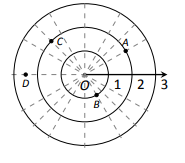9. Convert each of the following polar coordinates to rectangular, and each of the following rectangular coordinates to polar.
(a) $$A=P(2,\pi/4)$$
(b) $$B=P(2,-\pi/4)$$
(c) $$C=P(2,-1)$$
(d) $$D=P(-2,1)$$

10. Convert each of the following polar coordinates to rectangular, and each of the following rectangular coordinates to polar.
(a) $$A=P(3,\pi)$$
(b) $$B=P(1,2\pi /3)$$
(c) $$C=P(0,4)$$
(d) $$D=P(1,-\sqrt{3})$$

In Exercises 11-29, graph the polar function on the given interval.

11. $$r=2,\quad 0\le \theta\le \pi/2$$

12. $$\theta =\pi/6,\quad -1\le r\le \pi/2$$

13. $$r=1-\cos \theta,\quad [0,2\pi ]$$

14. $$r=2+\sin \theta,\quad [0,2\pi ]$$

15. $$r=2-\sin\theta,\quad [0,2\pi ]$$

16. $$r=1-2\sin \theta,\quad [0,2\pi ]$$

17. $$r=1+2\sin \theta,\quad [0,2\pi ]$$

18. $$r=\cos (2\theta),\quad [0,2\pi ]$$

19. $$r=\sin (3\theta),\quad [0,\pi ]$$

20. $$r=\cos (\theta /3),\quad [0,3\pi ]$$

21. $$r=\cos (2\theta /3),\quad [0,6\pi]$$

22. $$r=\theta /2,\quad [0,4\pi ]$$

23. $$r=3\sin (\theta),\quad [0,\pi ]$$

24. $$r=\cos \theta \sin\theta,\quad [0,2\pi ]$$

25. $$r=\theta^2-(\pi/2)^2,\quad [\pi,\pi ]$$

26. $$r=\frac{3}{5\sin \theta - \cos \theta},\quad [0,2\pi ]$$

27. $$r=\frac{-2}{3\cos \theta - 2\sin \theta},\quad [0,2\pi ]$$

28. $$r=3\sec \theta,\quad (-\pi/2,\pi/2)$$

29. $$r=3\csc \theta , \quad (0,\pi)$$

In Exercises 30-38, convert the polar equation to a rectangular equation.

30. $$r=2\cos \theta$$

31. $$r=-4\sin \theta$$

32. $$r=\cos \theta +\sin \theta$$

33. $$r=\frac{7}{5\sin \theta -2\cos \theta}$$

34. $$r=\frac{3}{\cos \theta}$$

35. $$r=\frac{4}{\sin \theta}$$

36. $$r=\tan \theta$$

37. $$r=2$$

38. $$\theta = \pi/6$$

In Exercises 39-46, convert the rectangular equation to a polar equation.

39. $$y=x$$

40. $$y=4x+7$$

41. $$x=5$$

42. $$y=5$$

43. $$x=y^2$$

44. $$x^2y=1$$

45. $$x^2+y^2=7$$

46. $$x+1)^2+y^2=1$$

In Exercises 47-54, find the points of intersection of the polar graphs.

47. $$r=\sin (2\theta) \text{ and }r=\cos \theta \text{ on }[0,\pi]$$.

48. $$r=\cos (2\theta) \text{ and }r=\cos \theta \text{ on }[0,\pi]$$.

49. $$r=2\cos \theta \text{ and }r=2\sin \theta \text{ on }[0,\pi]$$.

50. $$r=\sin \theta \text{ and }r=\sqrt{3}+3\sin \theta \text{ on }[0,2 \pi]$$.

51. $$r=\sin (3\theta) \text{ and }r=\cos (3\theta) \text{ on }[0,\pi]$$.

52. $$r=3\cos \theta \text{ and }r=1+\cos \theta \text{ on }[-\pi,\pi]$$.

53. $$r=1 \text{ and }r=2\sin (2\theta) \text{ on }[0,2\pi]$$.

54. $$r=1-\cos \theta \text{ and }r=1+\sin \theta \text{ on }[0,2\pi]$$.

55. Pick an integer value for n, where $$n\ne 2,3$$ and use technology to plot $$r=\sin \left ( \frac{m}{n}\theta\right )$$ for three different integer values of m. Sketch these and determine a minimal interval on which the entire graph is shown.

56. Create your own polar function, $$r=f(\theta)$$ and sketch it. Describe why the graph looks as it does.

## 9.5: Calculus and Polar Functions

### Terms and Concepts

1. Given polar equation $$r=f(\theta)$$, how can one create parametric equations of the same curve?

2. With rectangular coordinates, it is natural to approximate are with ________; with polar coordinates, it is natural to approximate area with _______.

### Problems

In Exercises 3-10, find;
(a)
$$\frac{dy}{dx}$$
(b) the equation of the tangent and normal lines to the curve at the indicated $$\theta$$-value.

3. $$r=1;\quad \theta =\pi/4$$

4. $$r=\cos \theta;\quad \theta =\pi/4$$

5. $$r=1+\sin \theta;\quad \theta =\pi/6$$

6. $$r=1-\cos \theta;\quad \theta =3\pi/4$$

7. $$r=\theta;\quad \theta =\pi/2$$

8. $$r=\cos (3\theta);\quad \theta =\pi/6$$

9. $$r=\sin (4\theta ) ;\quad \theta =\pi/3$$

10. $$r=\frac{1}{\sin \theta -\cos \theta};\quad \theta =\pi$$

In Exercises 11-14, find the values of $$\theta$$ in the given interval where the graph of the polar function has horizontal and vertical tangent lines.

11. $$r=3;\quad [0,2\pi]$$

12. $$r=2\sin \theta;\quad [0,\pi]$$

13. $$r=\cos (2\theta);\quad [0,2\pi]$$

14. $$r=1+\cos \theta;\quad [0,2\pi]$$

In Exercises 15-16, find the equation of the lines tangent to the graph at the pole.

15. $$r=\sin \theta ;\quad [0,\pi]$$

16. $$r=\sin (3\theta);\quad [0,\pi]$$

In Exercises 17-27, find the area of the described region.

17. Enclosed by the circle: $$r=4\sin \theta$$

18. Enclosed by the circle $$r=5$$

19. Enclosed by one petal of $$r=\sin (3\theta)$$

20. Enclosed by the cardiod $$r=1-\sin \theta$$

21. Enclosed by the inner loop of the limacon $$r=1+2\cos t$$

22. Enclosed by the outer loop of the limacon $$r=1+2\cos t$$ (including area enclosed by the inner loop)

23. Enclosed between the inner and outer loop of the limacon $$r=1+2\cos t$$

24. Enclosed by $$r=2\cos \theta\text{ and }r=\sin\theta$$, as shown:25. Enclosed by $$r=\cos (3\theta)\text{ and }r=\sin (3\theta)$$, as shown: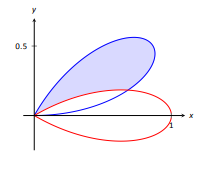26. Enclosed by $$r=\cos \theta \text{ and }r=\sin (2\theta)$$, as shown:27. Enclosed by $$r=\cos \theta \text{ and }r=1-\cos \theta$$, as shown: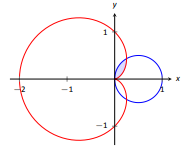In Exercises 28-32, answer the questions involving arc length.

28. Let $$x(\theta)=f(\theta)\cos \theta \text{ and }y(\theta)=f(\theta)\sin \theta$$. Show, as suggested by the text, that $$x'(\theta)^2+y'(\theta)^2=f'(\theta)^2+f(\theta)^2$$.

29. Use the arc length formula to compute the arc length of the circle $$r=2$$.

30. Use the arc length formula to compute the arc length of the circle $$r=4\sin \theta$$.

31. Approximate the arc length of one petal of the rose curve $$r=\sin (3\theta)$$ with Simpson's Rule and $$n=4$$.

32. Approximate the arc length of the cardiod $$r=1+\cos \theta$$ with Simpson's Rule and $$n=6$$.

In Exercises 33-37, answer the questions involving surface area.

33. Use Key Idea 44 to find the surface area of the sphere formed by revolving the circle $$r=2$$ about the initial ray.

34. Use Key Idea 44 to find the surface area of the sphere formed by revolving the circle $$r=2\cos \theta$$ about the initial ray.

35. Find the surface area of a solid formed by revolving the cardiod $$r=1+\cos \theta$$ about the initial ray.

36. Find the surface area of the solid formed by revolving the circle $$r=2\cos \theta$$ about the line $$\theta=\pi/2$$.

37. Find the surface area of the solid formed by revolving the line $$r=3\sec \theta,\,-\pi/4 \le \theta \le \pi/4$$, about the line $$\theta=\pi/2$$.